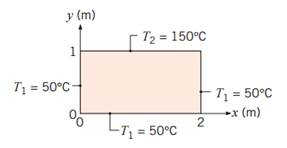### Create an Account

Home / Questions / A two dimensional rectangular plate is subjected to prescribed boundary conditions Using t...

# A two dimensional rectangular plate is subjected to prescribed boundary conditions Using the results of the exact solution for the heat equation presented in Section 42 calculate the temperature at

A two-dimensional rectangular plate is subjected to prescribed boundary conditions. Using the results of the exact solution for the heat equation presented in Section 4.2, calculate the temperature at the midpoint (1, 0.5) by considering the first five nonzero terms of the infinite series that must be evaluated. Assess the error resulting from using only the first three terms of the infinite series. Plot the temperature distributions T(x, 0.5) and T(1.0, y).Jun 28 2020 View more View LessSubscribe To Get Solution